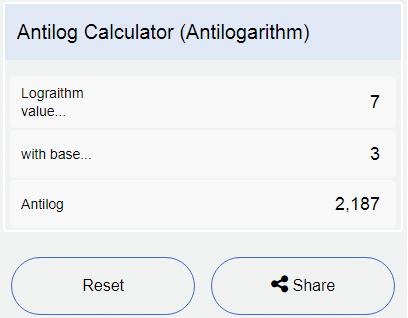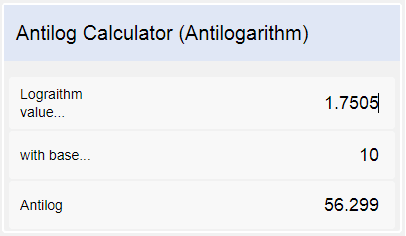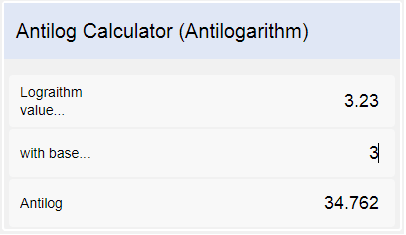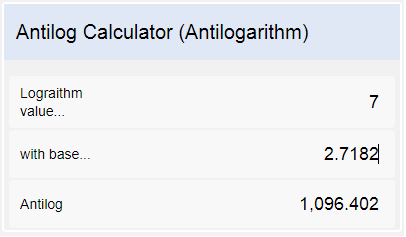# Antilog Calculator (Antilogarithm)

Antilog or antilogarithm is the inverse of the logarithm. In order to calculate the antilog of any number to any arbitrary base, an antilogarithm online calculator is a useful tool that is equally essential for teachers, students, scientists, and anyone who wishes to get quick antilog results.

The use of antilogarithms dates back to the sixteenth century when they were first discovered. But unfortunately, people then used complex tables to calculate the antilog of any quantity. Now that we have the online antilog calculators, the calculations are so much easy, hassle-free, and time-saving.

## How to Calculate Antilog Using CalculatorBeast?

Calculator Beast brings for its users an easy to use antilog calculator. It asks for nothing more than just adding the right values to the right column.

We have three sections in the calculator – the ‘Logarithm value’ section, where you will write the number whose antilog is required. Then we have ‘with base’ section, where you will write down the base of the logarithm, and in the ‘Antilog’ section, you will have quick, precise results up to 3 decimal places. It is just easy-peasy.

An illustration is shown for your convenience.### Concept of Antilogarithm with Solved Examples

Before we move to antilogarithms, let us look into logarithms.

Logarithms are pretty much related to exponents. Consider the following exponential or index form:

25 = 32

The above form can be written in a logarithmic form as:

Log2 32 = 5

Where 2 is the base, 5 is the index or exponent, and 32 is the answer to which the base 2 is raised to the power 5.

In general,

For any non-zero positive number a, except 1,

y = ax  <=> x = loga y

Now that the concept of logarithms is clear, we will discuss antilogarithms.

As the name suggests, antilogarithms are the inverse of logarithms. If log x = y, then x is called the antilogarithm of y. it is written as x = antilog y.

In the example discussed above, the log of 32 to the base 2, is 5, so the antilog of 5 to the base 2, is 32.

A few examples are illustrated with the help of our very straightforward antilog calculator to make sure you understand the use of it.

Example 1:

If log x = 1.7505, find the value of x

We already discussed that antilogarithms are the inverse of logarithms. So, to find the value of x, we need to find the antilog of 1.7505.

If log x = 1.7505

then x = antilog 1.7505

With our simple to use calculator, you just need to substitute 1.7505 in the ‘Logarithm value’ section. The base, when not mentioned, is always 10, and here you get the result, which is 56.299.Example 2:

Solve for x : log3 7 + log3 x = 5

Log3 x = 5 – log3 7

Calculate the value of log3 7 by using our online logarithm calculator and substitute above.

Log3 x = 5-1.77

x = (antilog)3 3.23

Substitute 3.23 in ‘Logarithm value’ section and 3 in the ‘with base’ section of calculator to get the value of x.

x = 34.762Example 3:

Solve for x : 2 lnx = 14

lnx = 7

x = (antilog)e 7

ln is the natural logarithm with base e which is 2.7182

x = 1096.402You can also write ‘e’ instead of writing 2.7182 in ‘with base’ section. Our antilog calculator understands that command.

Example 4:

Find the hydrogen ion concentration of the solution whose pH is 5.5.

The formula for pH of a solution is:

pH = –log[H+]

To find the hydrogen ion concentration, we can write the above formula as,

[H+] = antilog (-pH)

The value of pH is 5.5.

[H+] = antilog (-5.5)

Substitute the value in our calculator.

The hydrogen ion concentration of the solution is 0.00000316

### FAQs

#### 1. What are the most common bases used to calculate logarithms and antilogarithms?

The most common bases used to calculate logarithms and antilogarithms are base 10 and e.

Logarithms to the base 10 are known as Common Logarithms. They are often written as log10 or log or simply lg.

Logarithms to the base e are known as Natural Logarithms. They are often written as loge or just ln.

#### 2. How are logarithms and antilogarithms useful?

Logarithms and antilogarithms have many uses when it comes to real-life applications. Logs are often known as compressors because they are used to compress larger quantities into smaller.

Consider the following

102 = 100

103 = 1000

104 = 10000

105 = 100000

.

.

.

1012 = 1000000000000

Now consider graphing these numbers on a number line. There is such a huge difference between them that it seems almost impossible to graph them on a number line. So, what we do is simply consider the exponents 2, 3, 4, 5,… and plot them on a number line.

The same is the case with smaller numbers. Extremely smaller numbers are managed by the use of logarithms the way larger numbers are managed.

Nature is filled with innumerous larger or smaller numbers like the number of cells in the human body or the radius of an atom. These significantly larger or smaller numbers are difficult to handle while working on them. Logarithm compresses those numbers while antilogarithm converts back the numbers into the original form.

#### 3. What are the real-life applications of logarithms and antilogarithms?

There is an inevitable and massive use of logarithms and antilogarithms in real life. Some of the applications are:

1. The intensity of the earthquake is measured by a number on the Richter scale. The Richter scale is scaled to a base 10 logarithm. For instance, the 7.0 earthquake is 10 times stronger than the 6.0 earthquake and 1000 times stronger than the 4.0 earthquake.
2. Logarithmic functions are used to measure the acidity or pH of a solution. The basic formula for finding pH is –log[H+]
3. In astronomy, logarithms were used in the slide rules. It is the computation tool that helped men hit the moon.
4. Light sensitivity is logarithmic in nature. The amount of direct sunlight is 1 billion times brighter in nature than the light from a star in the night sky.
5. Logarithms are used to describe sound intensity in decibels. Every sound intensity can be classified in decibels according to a base 10 logarithmic scale. For instance, the normal conversation sound at 60 decibels has 10,000 times energy intensity than a whisper at 20 decibels.

The list is not just limited here. The use of logarithms is very wide in Number Theory, Probability Theory and Statistics, Psychology, and Astronomy. The antilogarithm, as discussed above, converts the compressed or shortened quantities to their original forms once the computations are handled by the effective use of logarithms.

#### 4. How do you calculate antilog of a number with base e?

The steps to calculate the antilog with natural base is as easy as finding the antilog with base 10 or any other base.

Imagine the logarithm number you want to calculate the antilog is 2.21. The natural base is an irrational constant, approximately equal to 2.7182. Substitute the numbers in respective columns, and our antilog calculator instantly solves for you giving you the result 9.115. Another example is illustrated above in the example section.

#### 5. How do you do antilog on a calculator?

You might have got confused many times after seeing that your calculator does not have an antilog function. Do not panic, it is there, but written in the exponent form like 10x. This function, usually, is the alternate of the log function in calculators. Even if you do not find it, or if the base of your logarithmic function is other than 10, simply write your log form into exponent form like y = ax and carry on with your calculations.

#### 6. What is the base of antilog?

The base of the logarithm is always a non-zero positive number other than 1. This can be seen in the logarithm definition box discussed above. Since antilogarithm is the inverse of the logarithm, it is calculated at the same base to which the logarithm of the respective number is given. For example,

Log2 x = 3

So the antilog will be

x = log2 -1 3

or x = antilog2 3

or x = 23

#### 7. What is the antilog of 3? Based on the explanation, what general statement can be given about calculating the antilogarithm of all real numbers?

The answer to this question varies with respect to the bases of the logarithm originally given. Consider the following logarithmic form,

Loga x = 3

So x is the antilog of 3, and it is written as,

x = antiloga 3

or x = a3

Now for different values of a, we will have different values of x.

Let us have some fun here. Substitute various values of ‘a’ i.e., the value in ‘with base’ section in our antilog calculator, and look at how drastically the result varies. For more fun, plot a graph and see if you could analyze any relationship or pattern.

Also, calculate the antilog of 3 with natural base e.

Now, based on the above explanation of calculating the antilog of 3, a general statement about the antilogarithm of real numbers is that they follow the same template as above. Their answers to antilog varies with respect to the bases.

#### 8. Can we calculate the antilog of negative numbers?

Antilog of negative numbers is calculated exactly in the same manner; antilog of positive numbers is calculated. Antilog of negative numbers is just the reciprocal of the base to which the power is raised. For instance,

Loga x = -3 , where a is a positive non-zero base and a>1

or, x = antiloga -3

or, x = a-3

or, x = 1/a3

Now simply by substituting the given value of the base in our antilog calculator, we can calculate antilog of -3 or any other negative number.

#### 9. What are mantissa and characteristic of a number?

To explain the characteristic and mantissa, we take the help of an example.

We know that log 1 = 0 and log 10 = 1. So, logarithm of any number that lies between 1 and 10 would be in between 0 and 1.

log 1 < log s <log 10

0 < log s < 1

Let us consider s = 5.57

0 < log 5.57 < 1

Since the log of 5.57 lies in between 0 and 1, we can say that

Log 5.57 = 0 + a positive decimal part

Now let us consider another case. We know that log 0.01 = -2 and log 0.001 = -3. So, logarithm of any number that lies between 0.01 and 0.001 would lie between -2 and -3.

log 0.001 < log s < log 0.01

-3 < log s < -2

We consider s = 0.0067

-3 < log 0.0067 < -2

Since the logarithm of 0.0067 lies between -2 and -3, we can say that

Log 0.00067 = -2 + a positive decimal part

From above example we state that logarithm of any number is made up of two parts. The integral part which can be either positive or negative is known as the characteristic and the decimal part which is always positive is known as the mantissa.

Log and antilog are interconvertible. Any form written in logarithm can be written in the antilogarithm form, and any antilogarithm form can be written in logarithm form.

y = axx = loga y , where a is a non-zero positive base and a>1

#### 11. What is ln? How do you calculate the antilog of any number with a natural base?

The ln is the logarithm to the natural base e, i.e., loge = ln, where e is an irrational constant equal to 2.71828… Natural logarithms are also known as Naperian logarithms. They are the inverse of the exponential function, which are extremely significant in finding the growth or decay of any process with respect to time.

Since we already know that antilogarithms are the inverse of logarithms, and as discussed above that natural logarithm is the inverse of the exponential function, so this clearly shows that antilog of the natural log or ln is simply the exponential function.

To calculate the antilog of any number with the natural base, simply write the number whose antilog is required in the ‘logarithm value’ section of our antilog calculator. Now in ‘with base’ section, type 2.71828, and there you have your required result.

#### 12. What is a googol?

US mathematician Edward Kasner came up with the concept of googol. Googol is a very large number 10100, i.e., the digit 1 followed by a hundred zeroes. It is a number that has no apparent significance in mathematics, but it is used for comparing very large quantities like the mass of the visible universe which is estimated to be around 1050 to 1060 kilograms or like the total number of elementary particles in the universe which are estimated to be 1080.

You must be wondering why I bought the subject of googol while talking about antilogarithms. Well, the googol is the value of antilog10 100. Interesting, right?